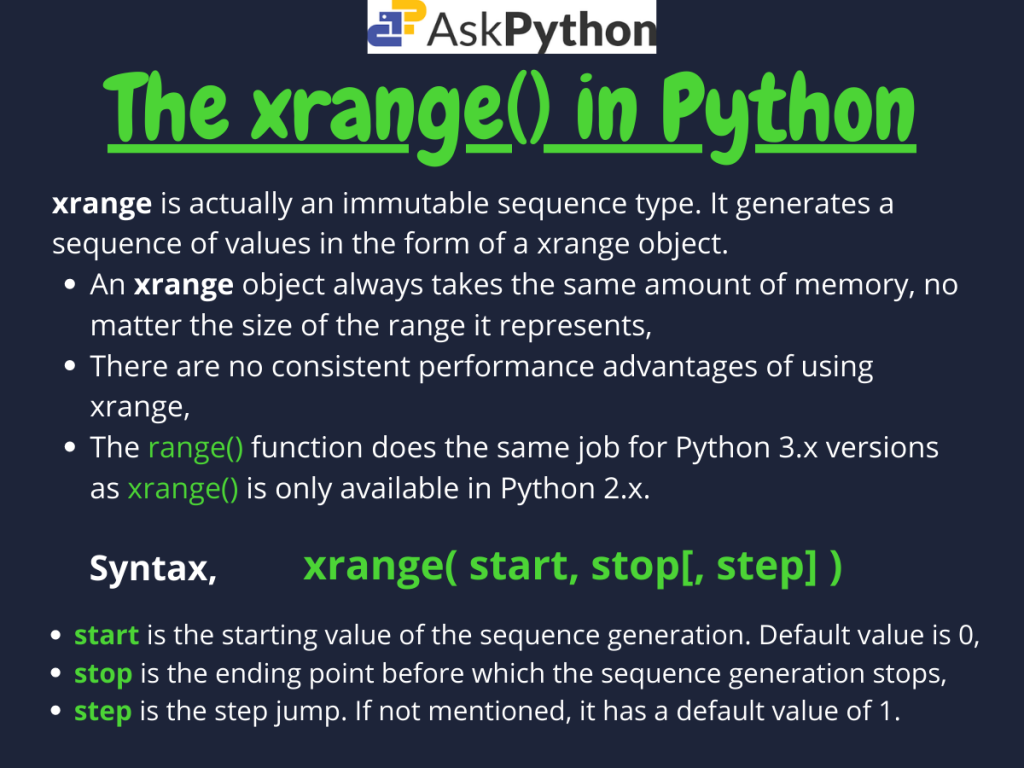# Understanding the Python xrange() MethodToday in this tutorial, we are going to discuss the Python xrange() method.

The `xrange()` method is only available for use in Python 2.x versions and used in loops for traversing or iterating through the sequence.

## Basics of the Python xrange() Method

The Python `xrange()` method returns a `xrange` type object which is an immutable sequence commonly used for looping. These objects have very little behavior and only support indexing, iteration, and the `len()` function.

```xrange(start, stop[, step])
```

Here:

• start(optional) is the starting point from which the sequence generation would start. It is included in the sequence and if not mentioned is by default set to 0,
• stop is the number before which the sequence generation would stop(exclusive),
• step(optional) is the step jump the function would to take while sequence generation or iteration. By default, it has a value of 1.

## Using the Python xrange() Method

Now let us look at the various ways we can actually use the Python `xrange()` method.

### 1. With only stop parameter

The two parameters, `step` and `start` are optional as they have default values of 1 and respectively. Hence we can use the Python `xrange()` method just by specifying the `stop` parameter.

When only stop is mentioned, the `xrange()` function creates a sequence ranging from 0 to (stop-1) with step 1. Look at the example below.

```# xrange() with only stop parameter

x = xrange(4)

print "Type of returned object = ", type(x)
print "Sequence generated by xrange() 1 parameter: ", list(x)
```

Output:

```Type of returned object =  <type 'xrange'>
Sequence generated by xrange() 1 parameter:  [0, 1, 2, 3]
```

Here, the type of sequence generated by the method is of type `xrange` as mentioned earlier. Type-casting the `xrange()` output into a list gives us a list containing the values 0 to 3(4-1) with step 1.

### 2. With start and stop parameters

Similarly, we can also use the Python `xrange()` method with two parameters. In this case by default, the `step` has a value 1.

```# xrange() with start & stop parameters

x = xrange(2, 9)

print "Type of returned object = ", type(x)
print "Sequence generated by xrange() 2 parameters: ", list(x)
```

Output:

```Type of returned object =  <type 'xrange'>
Sequence generated by xrange() 2 parameters:  [2, 3, 4, 5, 6, 7, 8]
```

As we can see from the above output, the Python `xrange` object this time contains values ranging from 2(start) to 8(stop-1) with a default step 1.

### 3. With all start, stop, and stop values

When all the parameters are mentioned, the Python `xrange()` function gives us a `xrange` object with values ranging from start to stop-1 as it did in the previous case. But this time, the jump from one element to another is equal to the `step` passed.

Have a look at the example below carefully. Here we have considered step as 5, start=5 and, stop=40.

```# xrange() with 3 parameters

x = xrange(5, 40, 5) #positive step
y = xrange(9, 1, -1) #negative step

print "Sequences generated for 3 parameters by xrange"
print "x : ", list(x)
print "y : ", list(y)
```

Output:

```Sequences generated for 3 parameters by xrange
x :  [5, 10, 15, 20, 25, 30, 35]
y :  [9, 8, 7, 6, 5, 4, 3, 2]
```

From the output, it is clear that the sequence is generated with values in range 5 to 39(40-1). For the last element, since 40 exceeds the `stop-1` mark it is not considered.

## Using Python xrange() in loops

As we mentioned earlier, `xrange()` is widely used in `for` loop structures in Python 2. For example in the code below we try to print the table for the number 10.

```# using xrange in loops

print "Table of 10: "

for i in xrange(10, 101, 10):
print i
```

Output:

```Table of 10:
10
20
30
40
50
60
70
80
90
100
```

Here, we have mentioned `start=10`, `stop=101` and `step=10`. Stop has been considered as 101 since we want the sequence to include the 100(100-1) element. As we can see we get all the multiples of 10 ranging from 10 to 100.

Hence, the output is justified.

## Python xrange() vs range()

Normally, `xrange()` is only used when the user wants his/her code to be only designed for Python 2.x versions as it is not available for Python 3.x versions. For Python 3 the `range()` method replaces the `xrange()` method instead.

The major difference between the two function is that, both of them return different objects with different properties.

The `xrange()` method returns a `xrange` object which is an immutable sequence and which only supports iteration, indexing and len function as mentioned earlier.

On the other hand, the `range()` method returns a list which supports major functions like slicing, pop() etc.

```# xrange() vs range()

x = xrange(1, 10)
y = range(1, 10)

print "Type(x) = ", type(x)
print "Type(y) = ", type(y)
```

Output:

```Type(x) =  <type 'xrange'>
Type(y) =  <type 'list'>
```

Moreover, `xrange` objects are more useful when we need to build code with minimal space complexity as it takes fairly constant memory size independent of the range of values it stores.

If you want to write a program that can be run or executed on both Python 2 and 3, using the `range()` method makes more sense and hence is recommended.

## Conclusion

So in this tutorial, we understood the working as well as use of the Python `xrange()` method.

For any further questions, feel free to ask them in the comments below.